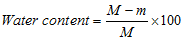# water content

(redirected from Volumetric water content)
Also found in: Acronyms, Encyclopedia.

## water content

Water in a contact lens expressed as a percentage of the total mass of the lens in its hydrated state under equilibrium conditions with physiological saline solution containing 9 g/l sodium chloride at a temperature of 20 ± 0.5ºC and with a stated pH value.where M is the mass of hydrated lens, m is the mass of dry lens.The US Food and Drug Administration (FDA) has categorized hydrogel contact lenses into four groups according to their water content and their surface reactivity (referred to as ionic if it contains more than 0.2% ionic material, and nonionic otherwise). Group 1: water content less than 50% and non-ionic. Group 2: water content greater than 50% and non-ionic. Group 3: water content less than 50% and ionic. Group 4: water content greater than 50% and ionic.
References in periodicals archive ?
This means that the product of the volumetric water content ([m.sup.3] solution [m.sup.-3] bulk soil) and the concentration of salt (mole salt [m.sup.-3] solution) is constant - this is the mass balance.
BD and all volumetric water content showed normal distribution of the data, except for those at 0.006 Mpa and 0.033 Mpa matric potentials.
Figure 11 presents plots of volumetric water content on the soil predicted by each ANN, one for each depth, based only on RFID tag and reader communication properties.
where [[theta].sub.s] is the saturated volumetric water content ([L.sup.3]/[L.sup.3]), [[PSI].sub.m] is the pressure head (suction) (L), [[theta].sub.f] is ([[theta].sub.s] - [[theta].sub.0]), and the subscript n means the n-layer.
Profiles for volumetric water content and electric conductivity as given by the sensor were reported.
Subsequently, the volumetric water contents of the different mixtures at each pressure head were determined from the dry bulk density and gravimetric water content.
To the improvement of this method in  a simplified model of the emulsion <<water--non-polar dielectric>> is proposed that allowed simply to determine the amount of volumetric water content in the mixture W as a function of dielectric permittivity [[epsilon].sub.2] mixture and dehydrated nonpolar liquid [[epsilon].sub.1] in the following form:
The volumetric water content (d) was obtained by multiplication of gravimetric water content (u) by bulk density p) of each soil i-th layer.
Therefore, to obtain a fundamental knowledge for estimating soil moisture with the PALSAR, we aimed in this study to establish a relationship between backscattering coefficients measured with the single polarization mode of PALSAR and volumetric water content, in small-scale farmlands.
Soil moisture had average condition at 0.230 [m.sup.3]/[m.sup.3] volumetric water content (VWC).
The content of readily available water (RAW) in each sample was decided through calculating the difference in volumetric water content held at -10 and -100 kPa (Fassman and Simcock, 2012).

Site: Follow: Share:
Open / Close